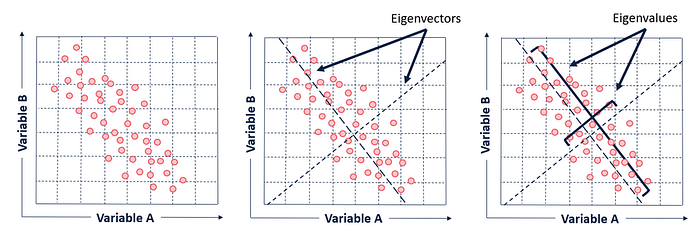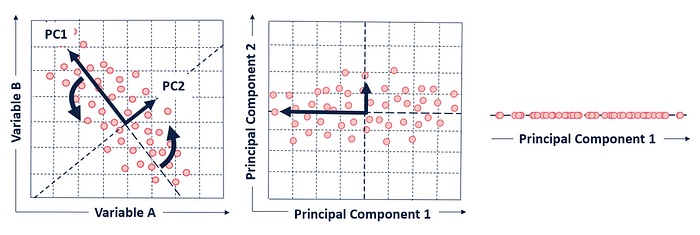Principal Component Analysis (PCA) is a very famous technique in Machine Learning. It is an unsupervised algorithm to reduce the number of dimensions in data, without losing the meaning of data. Reducing the number of dimensions makes it easier to understand and visualise the data and also compresses data, which in turn helps in faster processing. It has got a wide variety of applications in many fields like data analysis, computer vision and NLP. In this blogpost I’d like to give a brief overview into PCA and the basic math in it.

PCA is actually just a transformation method in linear algebra, which transforms the given data into combinations of original data in such a way that the new combinations will capture maximum variance in data while reducing the correlation between variables. Data is at first centered by subtracting the mean from each dimensions and then the covariance between each dimensions are calculated and arranged into a matrix called covariance matrix. Eigen vectors and eigen values are found for the covariance matrix and which is then used to find the transformed data with requred number of dimensions. Ok, so now let’s break this down into steps.

First step is to center data, that is to make the mean of data to zero. PCA without centering data might result in the first component corresponding with the mean of data rather than variance. For this all the values have the mean of the values subtracted from it.

Once the data is centered, no we need to find the covariance matrix. For that we need covariance between each dimensions. So what is covariance? For that let’s see what variance is.

Variance is a measure of spread of data in a dataset, with equation as:
$$s^{2}=\frac{ \sum_{i=1}^n \sqrt{\big( X_{i}- \overline{X} \big)^{2} } } { \big(n-1\big)}$$

Variance gives the spread of data in a single dimension, independent of other dimensions. Covariance gives how much the dimensions vary from the mean with respect to each other. The formula for covariance is very similar to the formula for variance. The formula for variance could be written like this: $$var(X)=\frac{ \sum_{i=1}^n \sqrt{\big( X_{i}- \overline{X} \big)\big( X_{i}- \overline{X} \big)} } { \big(n-1\big)}$$ similarly, the covarience of two dimensions X and Y is calculated as, $$covar(X,Y)=\frac{ \sum_{i=1}^n \sqrt{\big( X_{i}- \overline{X} \big)\big( Y_{i}- \overline{Y} \big)} } { \big(n-1\big)}$$ Covarience actually shows how the dimensions are related to each other. For example, considere a 2-dimensional data, with dimension H has the number of hours studied by a group of students and dimension M as the marks they recieved. A positive covarience value indicates that both marks and hous of study increases together, or in other words, as the hours of study increases, marks increases. Negative value of covarience indicates that the as the value of a dimension increases, value of other decreases. A zero value of covarience indicates that both the dimensions are independent of each other.

Next step is to make a covariance matrix out of the covariance values. Coaviance matrix will be a square, symmetric matrix of dimension nxn, where n is the number of dimensions. For a two dimensional data, the covariance matrix will be like: $$\begin{bmatrix}covar(x,x) & covar(x,y) \\covar(y,x) & covar(y,y) \end{bmatrix}$$ Diagonal values of the matrix will be variance value of each dimension. Because they are square and symmetrical, covariance matrixes are diagonalizable, which means an eigen decomposition can be calculated on the matrix. Next step is to find the eigen vectors and eigen values of the covariance matrix.
Considere a square matrix A, v is the eigen vector of if A[v] is a scalar multiple of v. ie., $$A.v = \lambda v$$ where λ is the eigen value.

In context of PCA, eigen vectors of covariance matrix are the axes of principal components in a dataset. Eigen vectors show the direction of principal components calculated by PCA, where eigen values show how stretched the axes are.A covariance matrix of n dimensions will have n pairs of eigen vectors and eigen values.
First eigen vector will be along the axis having the largest variance in data and the next one with lower variance and so on.
The eigenvalues are important because they provide a ranking criterion for the newly found axes. The principal components (eigenvectors) are sorted by descending eigenvalue. The principal components with the highest eigenvalues are “picked first” as principal components because they account for the most variance in the data.

Next step is to select the required number of eigen vectors, typically n-1 vectors with the highest eigen value. The selected eigen vectors are arranged into a feature vector. Transpose of the feature vector is then multiplied with transpose of the original data to get the new transformed dataset.Yeah, that’s it. we now have the new dataset with required number of dimensions. It is possible to get the old data back by just multiplying the the inverse of the feature vector with the new dataset, although there might a small loss in data.

PCA is a simple yet powerful tool. PCA is applied to compress images by reducing it’s dimensions. Most of the aappplication of PCA is around data visualisation as we are limited to represent data by 2 dimensions. It is effective at filtering noise and decreasing interdependency of variables. Many programming libraries have methods to apply PCA, so it’s not that important to know the programming side of PCA, but it is good to know theoretical side. There are many resources across internet, if you’d like to dive deeper into the maths of coding side of PCA.

Principal Component Analysis (PCA) from scratch in Python
A Tutorial on Principal Component Analysis has got detailed maths explanations.